# Section 2.2: Examples

## Equations in Engineering Problems

The following example show how the different categories of equation arise in various situations.

### Example 1: Simple Mixing Process

See Figure below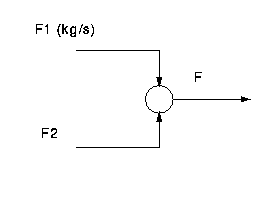Consider the behaviour of the process over a period of time when the two flowrates do not change, and any disturbances to the process resulting from previous changes in the flows have settled out.

total outflow rate = total inflow rate

F1 + F2 - F = 0

### Notes

• The condition of no change, etc, means that the process may be said to be at steady state.
• The properties of the process variables, i.e. the flows, are associated with one value at one point in the process, e.g. an inlet stream. There are a finite number of such points and in particular, not a continuum. This is called a lumped system.
• The equation is an algebraic equation, i.e. no integrals or derivatives.
• The solution is a number.

### Example 2: Tank Filling

See Figure below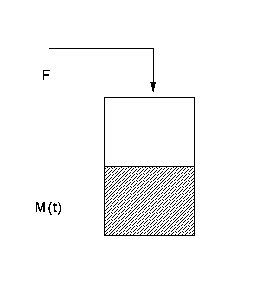Liquid flows at a rate F kg/s into a tank. The mass of material in the tank initially, at say time t=0 is M0 kg. There is no outflow from the tank.

rate of accumulation = net rate of inflow - net rate of outflow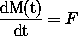With initial condition: M(t) = M0 at t=0

### Notes

• This is a dynamic situation because M changes with time.
• This is a lumped system because the property, holdup, is associated with a single point and is not distributed in space. That is, we can talk about the mass of material in the tank but not the mass of material at point x in the tank.
• The equation is an o.d.e. whose independent variable is time.
• The solution is functional variation of M(t).

### Example 3: Heat Transfer in a Slab

See Figure below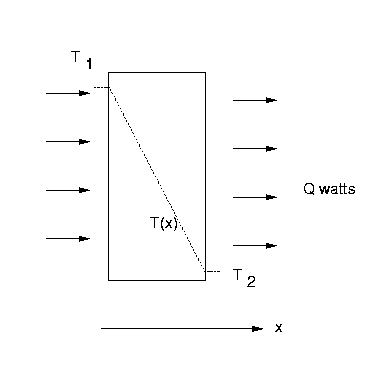Heat flows at a constant steady rate Q watts across a uniform slab. Each side of the slab is held at constant temperature. The temperature of the slab, of cross sectional area A and thermal conductivity k, varies across its thickness x and is denoted by T(x).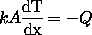### Notes

• This is called a distributed system because a property, the temperature of the slab, is distributed in space.
• There is one spatial dimension.
• There is no change in time because the heat flow is constant, thus this is once again a steady state situation.
• The equation describing the system is an ordinary differential equation.
• The independent variable in the o.d.e. is distance.
• The solution is the functional variation of the distributed property with distance, i.e. T(x).

### Example 4: Time Varying Convection by Plug Flow in a Pipe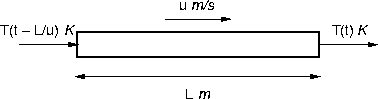This is described by a p.d.e. whose independent variables are time and distance along the pipe. Consider the situation where the temperature T is changing with distance x down a pipe of total length L where the velocity of flow is u.

This is described by the convective flow terms of the Navier-Stokes equation: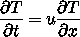Although this is a complicated looking equation it turns out to have a simple solution. The temperature of the fluid leaving the pipe at time t is exactly the same as the temperature entering the pipe at a previous time (t - L/u) since L/u is the residence time for the fluid in the pipe.

### More Complicated Situations

• Steady state systems in two or more dimensions are described by p.d.e.s whose independent variables are all the spatial dimensions involved.
• The unsteady state of distributed systems involves equations containing p.d.e.s with independent variables of both space and time.
• The solutions of p.d.e.s are functions or functionals.
• Distributed problems may contain integrals, leading to integral equations.

## Summary and Further Points

• Steady state lumped systems are described by algebraic equations
• Steady state distributed systems by o.d.e.s in distance
• Unsteady state lumped systems by o.d.e.s in time
• Anything more complicated by p.d.e.s.
• All these are different kinds of equations, requiring different solution methods.
• Algebraic equations also describe dynamic systems with no capacity and thus instant response.

Move back to Section 2 Index